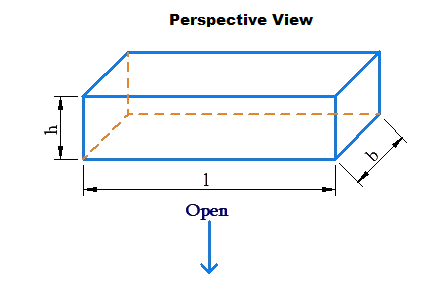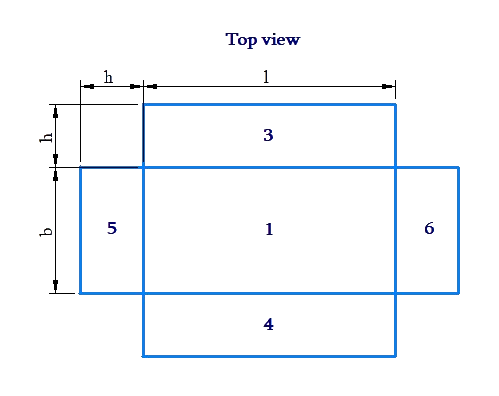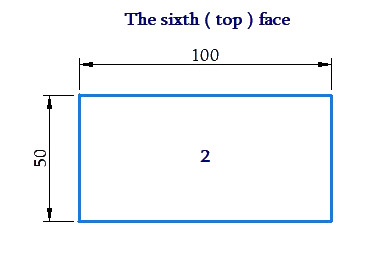# Surface Area of Cuboid

Go back to  'Mensuration-Solids'

Let us do a thought experiment, and open up a cuboid of dimensions $$l \times b \times h$$ so that we obtain six flat faces, as shown below:We have marked the six faces with numbers. What is the total surface area of the six faces? Clearly, it will be:

$\left( {2 \times l \times b} \right) + \left( {2 \times b \times h} \right) + \left( {2 \times l \times h} \right)$

Thus, the surface area S of any cuboid of dimensions  $$l$$, $$b$$ and $$h$$ will be:

$S = 2\left( {lb + bh + lh} \right)$

Note that the unit of measuring area will be the square of the unit of length. For example, if the dimensions have been measured in cm, the surface area will be obtained in cm2.

We can also talk about the lateral surface area of a cuboid. This is the combined surface area of the four vertical faces. Visualize a cuboidal room.

• The total surface area will be the combined area of the six faces of the room (the four vertical walls + the floor + the ceiling).
• The lateral surface area will be the combined surface area of the four vertical walls (the areas of the floor and the ceiling are not added).

For a cuboid with base dimensions $$l\times b$$ and height  $$h$$, the lateral surface area will be

$\left( {2 \times l \times h} \right) + \left( {2 \times b \times h} \right) = 2h\left( {l + b} \right)$

We note that for a cube of side $$l$$ units,

• Total surface area = $$6{l^2}\,{\rm{unit}}{{\rm{s}}^2}$$

• Lateral surface area = $$4{l^2}\,{\rm{unit}}{{\rm{s}}^2}$$

Mensuration and Solids
Mensuration and Solids
grade 10 | Questions Set 2
Mensuration and Solids
Mensuration and Solids
grade 10 | Questions Set 1